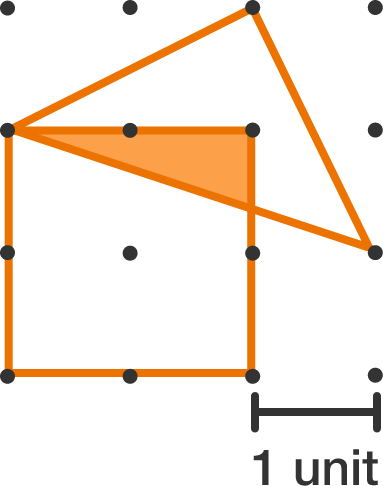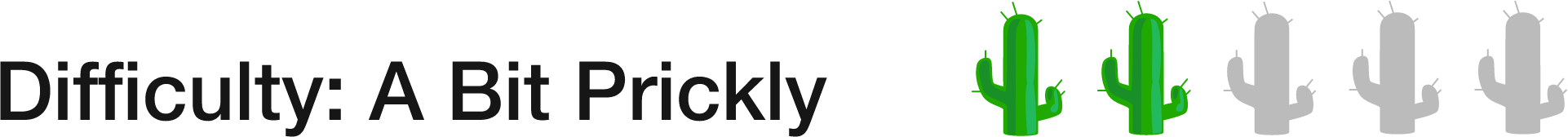# 27 of 100: Area Intersection Enigma

Geometry Level 1The dots in the grid above are all equally spaced. In square units, what is the area of the region shaded orange?There are many ways to solve this $-$ one of which involves finding the "coordinates" of that intersection point.

×